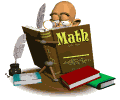•Welcome to Third Grade Honors Math

What is the Third Grade Honors Math Program?

The  third grade honors math program is an accelerated math program. It is a replacement program which means that students receive math instruction from Mrs. Nabozny on a daily basis and not from their classroom teacher. In this program, students will be taught a blend of the 3rd grade and 4th grade math curriculum. This means that by the end of the year, all 3rd grade skills and at least 75% of fourth grade skills have been taught. It is a fast paced program for our most able math students.

If students are taught most of 4th grade curriculum in 3rd grade, what happens in 4th grade?

Students who successfully complete the 3rd grade Advanced Math program move on to the 4th Grade Honors Math program, which focuses on 5th grade curriculum and beyond.

How do you cover two years of curriculum in one year?

Two years of curriculum in one year sounds excessive, and it would be if we taught every page of every lesson. We blended the curriculum of the two grade levels.  What this means is, for example, we teach the 3rd grade multiplication curriculum (basic concepts and mastering facts), and then move straight into the 4th grade skills of double digit multiplication, long division, area and perimeter. By doing this we eliminate a lot of repetition and revision at the start of each new skill set in fourth grade. The students in this program have proven themselves to be very strong math students who master skills quickly and can easily apply those skills without as much practice and repetition. Consequently not every question/page need be completed. The program is paced to suit the needs of the current students and so we can move through the curriculum much faster than the classroom  teachers.

What is the difference between Honors Math and Math Enrichment?

Honors math is a replacement program where students work with Mrs. Nabozny 5 days a week on the third and fourth grade math curriculum.
Math Enrichment is a once a week pull out program where we work on applying our math skills to more challenging problems. It involves a lot of higher level thinking and really helps students to develop their mathematical thinking and problem solving skills. Both programs provide mathematical challenges but in different formats.

What can you do to prepare for 3rd Grade Honors/Accelerated Math?

If you come into third grade with a good knowledge of multiplication facts, you will certainly feel a lot more comfortable once we start the multiplication units. It is not a requirement since multiplication will be taught, but it is something that will make life a whole easier :0)